• 9863020202
• contact@financeseva.in

## Capital Asset Pricing Model (CAPM)

Capital Asset pricing model (CAPM) is used to determine the current expected return of a specific security. This model assumes that every stock moves in some way relative to the market in general, and that by knowing this relationship, and the required rate of return for the market, and the minimum required risk free rate of return, the required rate of return can be determined for any stock. Use the capital asset pricing model calculator below to solve the formula.

### Capital Asset Pricing Model Definition

Capital Asset Pricing Model is used to value a stocks required rate of return as a function of its volatility, and the relative risk and rate of return offered by the market.

### Variables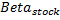=The relative volatility of a stock relative to the market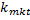=The rate of return of the market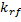=The risk free rate of return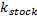=The required rate of return for a stock by investors

%
.
%

%

### Capital Asset Pricing Model Formula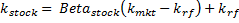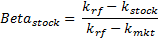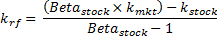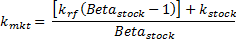#### CAPM Capital Asset Pricing Model

What is the CAPM Capital Asset Pricing Model?

The CAPM Capital Asset Pricing Model is a mathematical model intended to provide insight into how capital assets are priced in the market. The basic assumptions of this model give us insight into how it works. Investors are looking to generate a return on their funds by choosing assets to invest in with an expected rate of return. When investing these funds, investors put their capital at risk.

When deciding which asset to invest in, investors will look to compare the expected rates of return with the expected levels of risk. This means that all else being equal, investors will choose the asset that has the highest expected rate of return, and the asset that exposes them to the lowest level of risk possible.

The “market” is composed of all possible stocks, and is an aggregate of their returns and risk levels. When talking of risk in capital assets, we are really talking about their volatility. Volatility refers to the fluctuation in value of the asset, how much the price increases or decreases. A high degree of volatility means that a particular asset (stock) will increase and decrease in price to a higher degree than another asset with less volatility.

The CAPM Capital Asset Pricing Model assumes that the volatility of any one asset can be related to the volatility of the market as a whole. The relationship between the price of any one asset and the value of the market is known as Beta. An asset with a high Beta will increase in price more than the market when the market increases, and decrease more than the market when the market decreases. A Beta with a value of 1 is expected to move to the same degree as the market, a Beta with a value lower than 1 will move to a lesser degree than the market, and a Beta of greater than 1 will move to a higher degree than the market when the market moves. It is even possible to have a negative Beta. A negative Beta means that the price of the asset tends to decrease when the value of the market increases. As with the positive Beta, the greater the value of the negative Beta will indicate the degree to which the asset fluctuates inversely to the market.

The CAPM Capital Asset Pricing Model assumes that investors will never accept higher levels of risk (volatility) unless they are to be compensated with a higher expected rate of return. This follows form the basic assumption that the value of the market in the long run will increase. An asset with a Beta greater than one will expose the investor to higher levels of volatility, but will also reward the investor as the value of the asset will grow at a higher rate than the market.

The CAPM Capital Asset Pricing Model also assumes that investors can always invest in a risk free asset, which will offer them the minimum possible rate of return for any investment. In the real world, this tends to be based on the rate offered by federal government short term treasury bills, as these are considered to be the closest possible to risk free investments.

Since an investor will always want to expose themselves to the minimum level of risk, they must receive a premium rate of return relative to the amount of risk they are exposed to in any one asset. This risk premium, which is over and above the risk free rate of return, is the required rate of return that an asset must generate in order for anyone to invest in it. If the asset does not produce the required rate of return, then the price is too high relative to the risk exposure.

The CAPM Capital Asset Pricing Model concludes that this is the primary determinant of asset prices in the market. Other models follow this theory, such as the Gordon Model (Constant Dividend Growth), which assumes that price can be determined by the rate of return received by an investor.

What is the Risk Free Rate of Return?

The risk free rate of return in the CAPM Capital Asset Pricing Model refers to the rate of return an investor can receive without exposing their funds to any risk. Typically based on the rate paid on short term federal treasury bills, this interest rate forms the basis for the required rate of return on all assets.

No matter what the capital asset, the risk free rate of return will be included in the required rate of return. Any return over the risk free rate will be due to the risk premium required by investors to accept the higher level of risk in their portfolio.

The risk premium is an important component of the CAPM Capital Asset Pricing Model. The primary determinant of the required rate of return is the risk premium. Investors have a large selection of capital assets to invest in, which in aggregate compose the market. If an investor invested in the entire market, then their required rate of return would be the rate of return that the entire market returns to investors. The difference between this rate of return and the risk free rate would be the risk premium.

Since the volatility each asset can be compared against the volatility of the market, then it follows that their risk premium would adjust by the same degree. Since the total required rate of return is the sum of both the risk free rate and the risk premium, variation in the total required rate of return will be greater for high risk assets compared to low risk assets.

What is the Required Rate of Return?

The required rate of return is simply the sum of both the risk free rate and the risk premium. Each asset in the market will have a required rate of return, which can be used to determine the price of the asset. Required rate of return is always relative to the market rate of return, since it varies according to the volatility of each asset in the market.

What is Beta?

Beta is an important concept to the CAPM Capital Asset Pricing Model, since it determines the risk premium of any one asset relative to the risk of the market as a whole. Beta is a direct correlation of volatility between the market as a whole and any one asset. Is an asset increases at a rate of 200% of the market increase, then the Beta of that asset would be 2. A simple concept that shows the importance that investors place on risk, and the premium that they require in order to be willing to accept it.

How do you calculate the CAPM Capital Asset Pricing Model?

The CAPM Capital Asset Pricing Model can be calculated fairly simply. The CAPM Capital Asset Pricing Model Calculator above can be used to determine the required rate of return for any asset. The CAPM Capital Asset Pricing Model formula is as follow, and each variation of the formula is provided above next to the CAM Capital Asset Pricing Model Calculator.

The CAPM Capital Asset Pricing Model Formula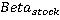=The relative volatility of a stock relative to the market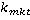=The rate of return of the market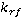=The risk free rate of return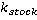=The required rate of return for a stock by investors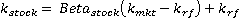As you can see, the required rate of return for any stock is composed of the two elements, the risk free rate and the risk premium. Once the values for the risk free rate and the market rate of return are determined, the required rate of return for all stocks can be easily determined simply by finding the Beta for each stock.

The CAPM Capital Asset Pricing Model Securities Market Line

The CAPM Capital Asset Pricing Model formula can be plotted on a graph, to produce the securities market line. The graph will have return on the vertical axis, and risk in the form of Beta on the horizontal axis. A securities market line following from the formula will produce a line intersecting the vertical axis at the risk free rate of return, and rising relative to the risk premium required by investors.

As investors become more risk averse, they require a higher risk premium to accept risk in their investments, and the slop of the securities market line increases. If the risk free rate of return changes, then the line shifts vertically by the same amount as the change in the risk free rate.

The theory of the securities market line is that all securities prices will fall along this line at their equilibrium. Since the price of a security is determined by the relative buy and sell orders in the market, a security that falls above the securities market line will be priced to low, relative to its risk/return characteristics. Investors will look to buy this security since the return is higher than the required risk premium. This inflow of buyers will increase the price of the security, reducing the expected return, until the expected return approaches the securities market line. At this point a security is at equilibrium, as the forces that would push it above or below the securities market line are balanced.

How to Use the CAPM Capital Asset Pricing Model Calculator

The CAPM Capital Asset Pricing Model Calculator above can be used to solve for any variable in the formula. Simply input the know variables to solve for the unknown. The result of the CAPM Capital Asset Pricing Model Calculator can be used in other valuation calculators, like the Gordon Constant Growth Model Calculator, to determine the actual price of a security.